# CenterNet: Objects as Points — A Comprehensive Guide

## A detailed technical breakdown on interesting research of CenterNet: Objects as Points

CenterNet: Objects as Points¹ is interesting research which disrupts one of the hot topics of Anchor Free Object Detection. We will be doing an exhaustive study of this paper with a concise modularized paradigm so that it will be easy for you to relate this article with an original research paper.

Also, I strongly urge you to go through the basics of Focal Loss before reading this blog, as it will help you to doubtlessly acknowledge the algorithmic flow of loss calculation used in this proposed method. You can get a detailed explanation of Focal Loss from this link.

Below given table of content will be followed from the beginning. If you want to jump to a specific part in this article, you can do that for easy readability.

# 1. Introduction

• There are two types of methods to regress and classify bounding box around an object in Anchor Free Object Detection approaches. i) Keypoint based approach ii) Center-based approach.
• The former predicts predefined key points from the network which are then used to generation of the bounding box around an object and classification of the same. CornerNet², CenterNet: Keypoint Triplets³ and Grid- RCNN⁴ are some networks using keypoint based approaches.
• The latter uses center-point or any part-point of an object to define positive and negative samples(instead of IoU..!) and from these positives, it predicts the distance to four coordinates for the generation of a bounding box. Some of the networks using this approach are FCOS⁵, DenseBox⁶, FSAF⁷, etc. They have their peculiar methods to generate positive samples and use that for regression of boxes, objectness, and class probabilities.
• CenterNet: Object as Points¹ follows the former viz. keypoint based approach for object detection. It considers the center of a box as an object as well as a key point and then uses this predicted center to find the coordinates/offsets of the bounding box.
• In this paper, a center prediction is considered as a standard keypoint estimation problem. After passing an image through Fully Convolutional Network, the final feature map outputs heatmaps for different key points. Peaks of these output feature maps are considered as predicted centers.
• Additionally, the network predicts the width and height of the box for these centers and each center will have its unique box width and height. This tightly coupled property helps them to remove the Non-Maximal Suppression step in post-processing.
• For classification, these heatmap peaks are also linked to a particular class to which it belongs to. So using these centers, dimensions, and class probabilities, object detection task is achieved.

# 2. Proposed Algorithm

Let’s divide this part into different subcategories and go step-by-step which will help you in both technical understanding and code implementation.

## 2.1 Overall Novel Workflow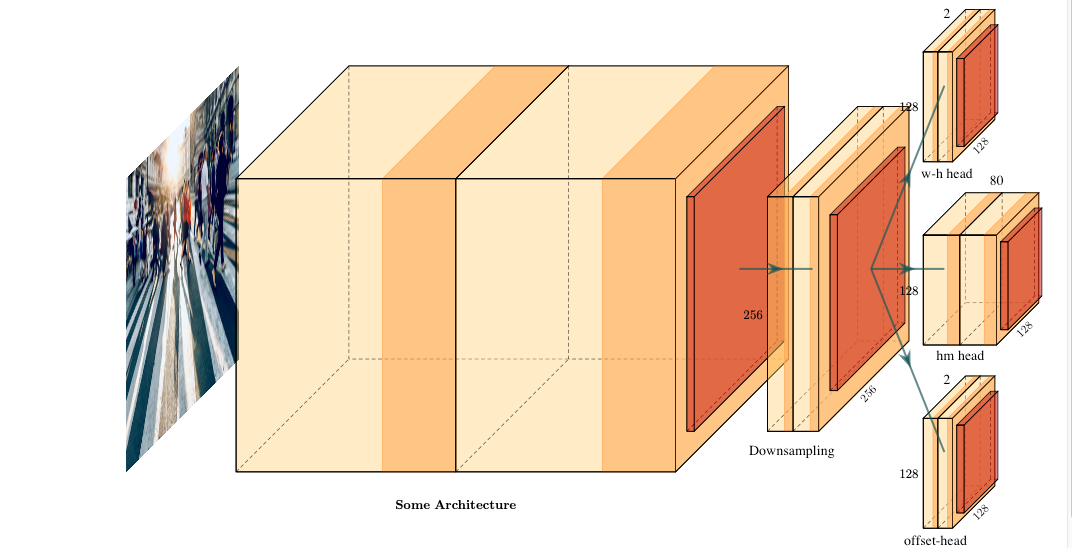Fig 1. Three heads are predicted after one forward pass from the network architecture. 1)Offset Head. 2) Heatmap Head. 3) Dimension Head. Here Some Architecture(FCN) referees to any of the feature extractors which we want to use(Specified heads are for object detection).
• Consider an input image I having width and height as W and H respectively(No of Channels are 3). R is an output stride that will decide the final dimensions of the given heads. All the heads will have the same height and width(H/R, W/R) but will have different C values. So the final head dimensions will be (W/R, H/R, C=[Classes/2/2])(As shown in Fig. 1, input dimensions are 512X512 and head dimensions are 128X128 considering stride R=4). Three heads considered as shown in Fig. 1. I) Heatmap Head II) Dimension Head III) Offset Head.

• This head is used for the estimation of the key points given an input image. In the case of object detection, keypoints are the box center. We have to predict heatmap Y_hat of dimensions (W/R, H/R, C), where R is the output stride, and C is the number of classes(80 in case of COCO Dataset). Here Y_hat is the function of x, y, c. A prediction Y_hat(x, y, c) = 1 corresponds to detected center for that particular class c. Y_hat(x, y, c) = 0 is considered as background.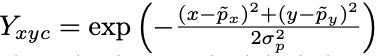Fig. 2 Gaussian Kernel used to splat box centers.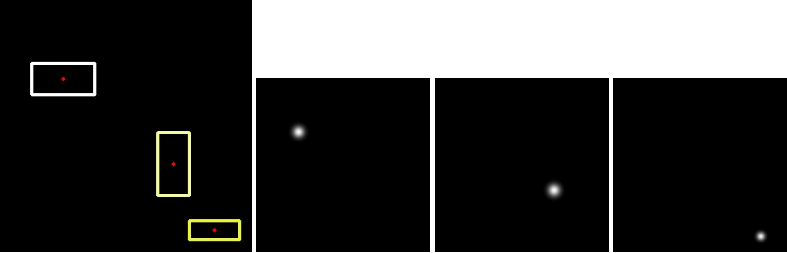Fig. 3(Left) Ground Truths of different classes(Represented in different colors). (Right) Three centers of respective classes splat into heatmaps using Gaussian Kernel.
• To form ground truth heatmaps for loss propagation, these centers are splat using Gaussian Kernels after converting them to low-resolution equivalent(In our case division by stride R. Denoted as p~). For example, If we have three classes(C=3) and input image dimensions are 400X400, then with a given stride(R=4), we have to generate 3 heatmaps(Each heatmap corresponding to a given class) of dimensions 100X100 as shown in Fig. 3. σ used in the kernel is the object-size adaptive standard deviation.
• If in a case two gaussian of the same class are overlapping, they take element-wise maximum to find the target class.

• This head is used for the prediction of the dimensions of the boxes viz. width and height. Given box coordinates, (x¹, y¹, x², y²) of object k and class c, they regress object sizes s_k = (x²-x¹, y²-y¹). This is achieved by solving a standard L1 distance norm. Dimensions of this heatmap are (W/R, H/R, 2)(w an h are predicted width and height of the box). To reduce the computational burden, they use single sized heatmaps for all object categories.

• This head is used to recover from the discretization error caused due to the downsampling of the input. After the prediction of the center points, we have to map these coordinates to a higher dimensional input image. This will cause a value disturbance as the original image pixel indices are integers and we will be predicting the float values. So to solve this issue they predict the local offsets O_hat. These local offset values are shared between objects present in an image. Dimensions of this head are (W/R, H/R, 2)(x and y are the coordinate offsets).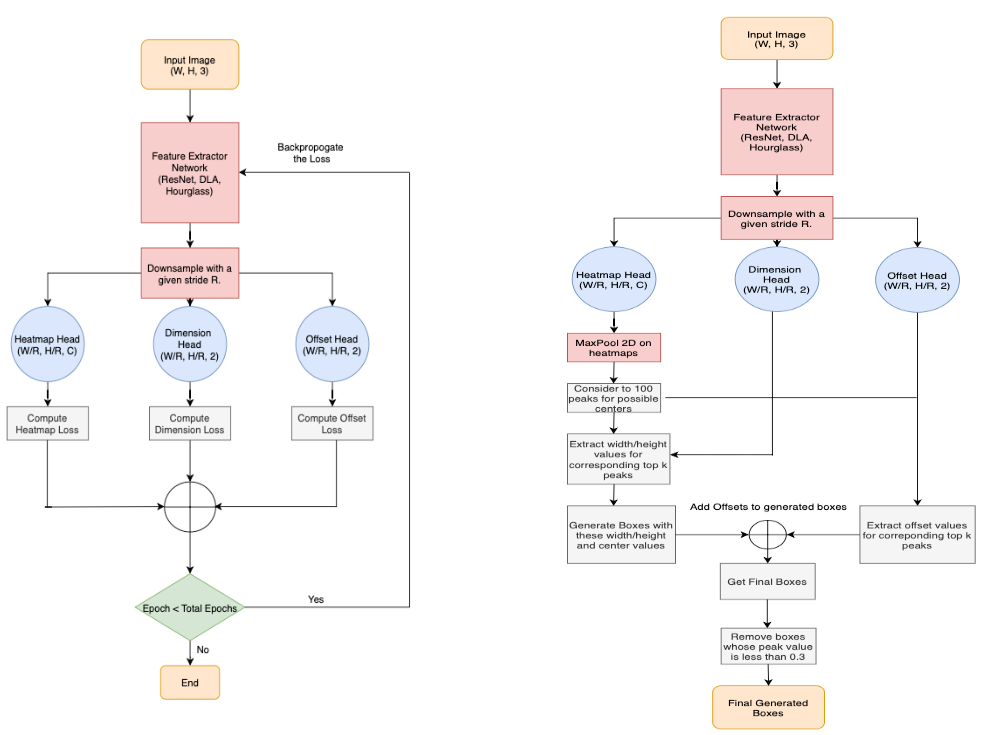Fig. 4 (Left) Training, (Right) Inference flowchart of the proposed Object Detection algorithm

## 2.2 Feature Extractor/ Backbone Used

• Four different feature extractors were used for the experiments. ResNet18, ResNet1⁰¹⁸, Deep Layer Aggregation Networks(DLA)⁹, and Stacked Hourglass Networks¹⁰. ResNets and DLA were modified by adding Deconvolutional and Deformable Convolutional Layers.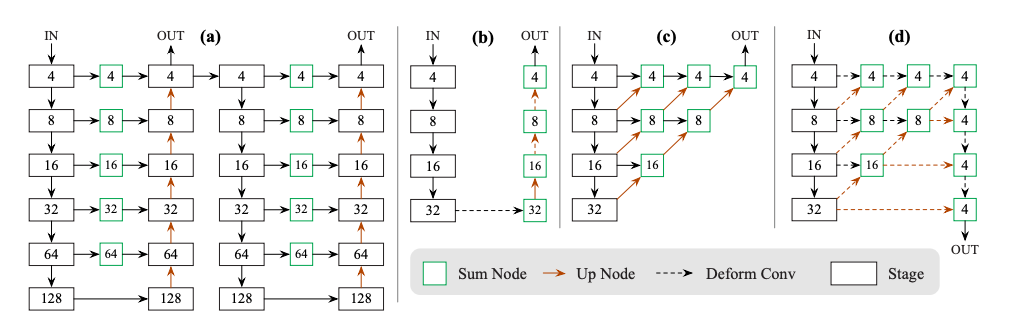Fig. 5 Modifications done in network architectures. (a) Stacked Hourglass Network. (b) ResNets with Transposed and Deformable Convolution layers. © Original DLA-34. (d) Modified DLA-34 by adding skip connections and Deformable Convolutional Layers.

— Hourglass Module

• The stacked Hourglass Network downsamples the input by 4×, followed by two sequential hourglass modules. Each hourglass module is made up of a uniform chain of 5-layer down- and up-convolutional network with skip connections. No changes were made in this network.

— Modified ResNet Modules

• Standard ResNet modules are augmented with three transposed convolutional networks to incorporate higher resolution outputs.
• Some modifications are by reducing the output filters of upsampling layers to 256, 128, and 64 respectively for computational reduction. The addition of a 3X3 deformable convolutional layer between each upsampling layers helped to get decent results on some standard datasets.

— Modified DLA -34

• DLA is a classification network with hierarchical skip connections as shown in Fig. 6(c). These upsampling layers are replaced by deformable convolutional layers and some other augmentations of deformable skip connections from lower layers(high-resolution feature maps) to output helped to increase the feature map resolution symmetrically. As seen in Fig. 6(d), 3X3 deformable layers are replaced with simple convolutions in every upsample layer depicted in normal DLA-34.

## 2.3 Loss Calculation and Propagation

• Here comes the main part of the algorithm. Once heatmaps are generated from the network, how will you propagate the loss for stabilized and legit training? In this paper, they used straightforward but effective loss functions to overcome and balance the bias between the training of different heads mentioned in 2.1.
• Three Loss functions are mentioned in the given paper¹. 1) Heatmap Variant Focal Loss, 2) L1 Norm Offset Loss 3) L1 Norm Dimension Size Loss.

— Heatmap Variant Focal Loss

• Before reading this, I urge you to refresh the basics of Focal Loss. Here Loss Function is divided into two parts of positive and negative samples as shown in Fig. 6.

— When Y = 1

• When predicted Y_hat is close to 1, let’s say 0.95, it considers as an easy example(well-classified example) and so by the logic of Focal Loss it will decrease the weightage of the propagated loss.
• The same logic is followed for hard examples(misclassified example) but instead of decreasing the weight, it will increase the slope of the value by parameter α. Here α is set to 2.

— When Y != 1(Otherwise)

• When predicted Y_hat is very close to 0, say 0.005, then (Y_hat)^α will make the overall loss zero, and less weight will be assigned to the propagated loss as stated in the premise of Focal Loss.
• Now what if Y_hat is not very close to 0 and has a value near to 1, but in the vicinity of our ground truth heatmap. Here comes the beauty of this loss.
• We know that our ground truths are the gaussian kernel outputs. So there is no sudden drop in the values near Y=1. It considers values lying inside this gaussian outputs as candidate positives. Let’s understand with an example.
1. We got the predicted Y_hat as 0.9 and is near to the center point peak of ground truth. Here there is a misclassification as value should be very very near to 0 according to simple logistic regression loss logic. But as predicted Y_hat is close to 1, the loss propagated will be less weighted even in a condition of misclassification as the loss will get compensated due to the term (1-Y)^β(Value of Y will be close to 1 in a region near center peak).
2. We got predicted Y_hat as 0.9 and is far from the center point peak. Now in this condition of misclassification, a large loss will be propagated due to term (1-Y)^β as it does not lie in that splatted region, and the value of Y will be very close to 0. Here β is set to 4.

If you have got the taste of this variant loss, you can observe one thing. The design of this loss helps to increase the number of positive examples by considering the heatmap values generated by gaussian kernels which further helps to decrease the bias between positives and negatives.

— L1 Norm Offset Loss

• This is a simple L1 Norm of the predicted offset O_hat and the ground truth offset values.
• What are ground truth offset values? Let’s say you have a center point at (18, 22) in an original high-resolution image. Now when downsampled, with stride = 4, the mapped coordinates will be (4, 5) on a low-resolution feature map. As you can see, there is an offset error of 0.5 in both cases.
• In the case of keypoint estimation, it becomes important to handle this issue, as keypoints are very position sensitive. To solve this, offset loss function is added to for obtaining more accurate results.

This supervision only acts at the position of key points, all other locations are ignored.

— L1 Norm Dimension Size Loss

• Regression of the width and height of bounding boxes is done using standard L1 Norm Loss of the predicted and ground truth width-height coordinates. Here s_hat are the predicted dimensions and s are actual ground truth sizes.
• Raw pixel values are used to calculate the loss instead of normalizing with the feature map size.
• Total Loss propagated by the network is shown in Fig. 9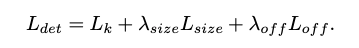Fig. 9 Total Loss of CenterNet: Objects as Points. λ_size = 0.1/ λ_offset = 1.

## 2.4 Training/Inference

— Training

Below given is the training snippet mentioned by authors. Other hardware related information can be found in the Training section of the paper.

`###Training Specification###Data Augmentation: Random Flip, Random Scaling: [0.6, 1.3]Input Resolution: 512X512Stride R = 4Output Resolution: 128X128Optimizer: AdamDataset: COCO, PascalVOC`

— Inference

• Some use cases are stated in the given paper.
1. Object Detection
• At inference time, peaks of the heatmaps are calculated by seeing the maximum value near the 8-pixel neighborhood in a heatmap and keeping the first 100 peaks of all the different classes independently. This operation is achieved by 3X3 MaxPool Operation on the obtained feature map.
• The obtained peak coordinates are used to get the dimensions and offset predictions. You can get to know this part better by going through this piece of code.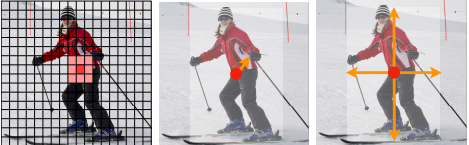Fig 10. (Left) Keypoint Heatmap. (Middle) Keypoint Offsets. (Right) Dimensions of box¹

2. Pose Estimation

• Pose estimation is considered a simple keypoint estimation problem. Here instead of 80 as a value of C, k = 34 is used(In Case of COCO Dataset: 17 key points). These offsets are predicted for each keypoint directly regressing from the centers.
• To refine keypoint estimation, k heatmaps are also predicted using the approach discussed in the case of Object Detection and Variant Focal Loss/Offset Loss.
• Decoding the output heatmaps, offsets, and keypoints is given in this link.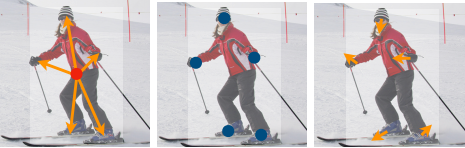Fig. 11 (Left) Keypoint Center Offsets. (Middle) Keypoint Heatmaps. (Right) Offsets of keypoints¹

# 3. Results/Comparisons

## 3.2 Comparison of speed and mAP of object detection results between different state-of-the-art methods.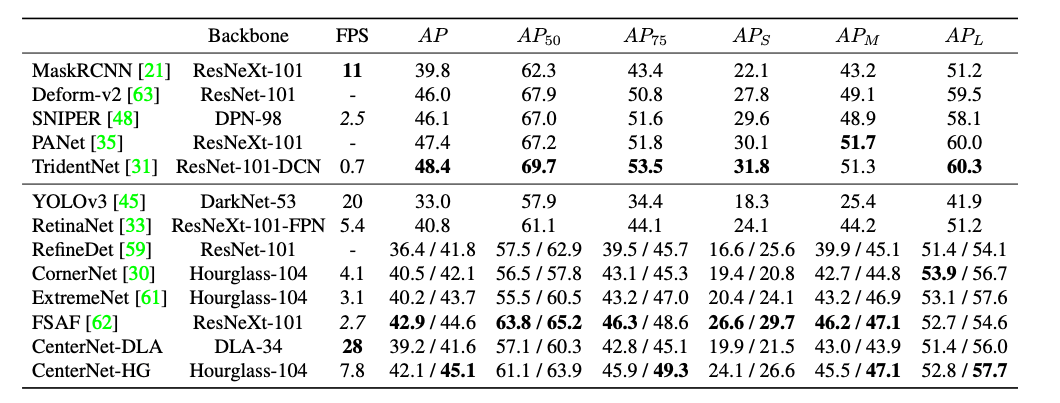Fig. 13 Comparison of CenterNet with different present methods on COCO Dataset¹

# 4. Conclusion

• CenterNet: Objects as Points¹ proposes a fast, simple, and accurate method for predicting the bounding boxes and poses of objects and persons respectively without the use of different NMS threshold and post-processing.
• They also have mentioned about the 3D object detection task by predicting depth factor from the images and have wide use cases in the field of machine vision(This part is not mentioned in this article).
• Some challenges like points collision were faced during the implementations but with the given supposed solutions, it didn't affect that much to the accuracy results.

# 5. Extra References Related To The Paper

 CenterNet: Objects as Points: [paper] [github]

 ResNets101: Deep Residual Learning for Image Recognition

DLA: Deep Layer Aggregation

 Hourglass Networks: Stacked Hourglass Networks for Human Pose Estimation

Deformable Convolutional Networks

Focal Loss for Dense Object Detection

Other Implementations of this paper in different frameworks: [codebase 1][codebase 2]

Thank you for reading the article. I hope as a writer I was able to convey the topic with utmost clarity. Please leave a comment if you have any feedback/doubts.

A clap would be wonderful feedback too.

PS: I am trying to make research ideas available to all and it would be a great help if you can spread the word out by sharing and following.

--

--

--

## More from VisionWizard

A Medium publication for curated information of best research ideas in the field of AI.

## Parts of Speech Tagging with Hidden Markov Model## Linear Regression : decoded## Deploy image segmentation (Mask R-CNN) model service using TensorFlow Serving & Flask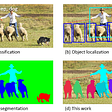## What are radiological deep learning models actually learning?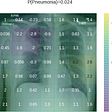## Customizing the SentenceDetector in Spark NLP## Review: Swin Transformer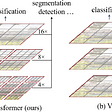## Shreejal Trivedi

Deep Learning || Computer Vision || AI || Editor — VisionWizard

## AI Tennis Ball Bounce Detection## Object Detection: R-CNN & Fast R-CNN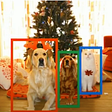## Monitor Social Distancing using Python, YOLOv5, OpenCV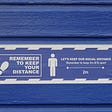## YOLO v3 Explained